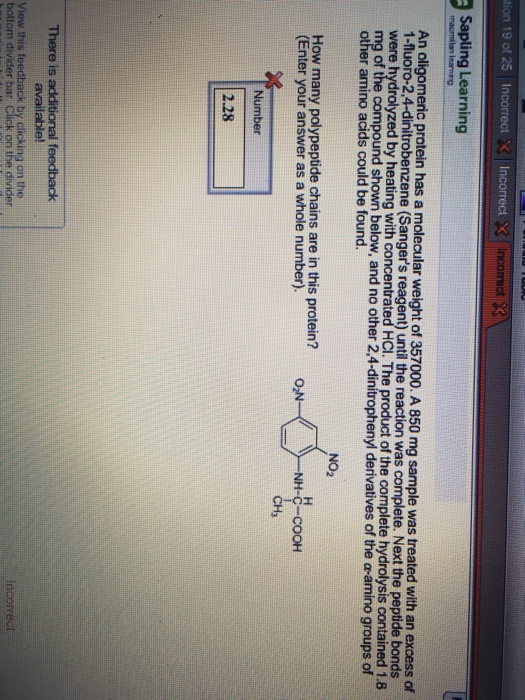# Question & Answer: An oligomeric protein has a molecular weight of 357000. A 850 mg sample was treated with an…..An oligomeric protein has a molecular weight of 357000. A 850 mg sample was treated with an excess of 1-fluoro-2, 4-dinitrobenzene (Sanger’s reagent) until the reaction was complete. Next the peptide bonds were hydrolyzed by heating with concentrated HCl. The product of the was complete hydrolysis contained 1.8 mg of the compound shown below, and no other 2, 4-dinitrophenyl derivatives of the alpha-amino groups of other amino acids could be found. How many polypeptide chains are in this protein? (Enter your answer as a whole number).

the molecular weight of the sample = 357000 mg/mmol

Calculate the molecular weight of protein as shown in the figure = C9N3O6H9 = 9*12+3*14+16*6+1*9 = 108+42+96+9

Don't use plagiarized sources. Get Your Custom Essay on
Question & Answer: An oligomeric protein has a molecular weight of 357000. A 850 mg sample was treated with an…..
GET AN ESSAY WRITTEN FOR YOU FROM AS LOW AS \$13/PAGE

= 255 mg/mmol

m moles of the compound protein shown in the figure =

=1.8 mg/255 mg/mmol = 0.00706 mmoles

mmoles of the sample = 850 mg/357000 mg/mmole

= 0.00238 mmoles

Polypeptide chains in the Protein = mmoles of protein / mmoles of sample

= 0.00706 / 0.00238 = 2.97

In the whole number, polypeptide chains = 2

Since the calculation is very sensitive in integers, then 2.97 can also be considered as 3. However the perfect answer is 2.